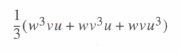In general, Mathcad’s symbolic processor and Mathcad’s numerical processor don’t communicate with one another. Because of this, it’s possible to set up a complicated numerical calculation without knowing that you could have reduced it to an equivalent but much simpler problem by judicious use of the symbolic processor.

You can, however, make the numerical processor ask the symbolic processor for advice before starting what could be a needlessly complex calculation. In effect, the symbolic processor acts like the numerical processor’s consultant, examining each expression and recommending a better way to evaluate it whenever possible. It does this for each expression in the worksheet except for those you specifically tell it to ignore.

For example, if you were to evaluate an expression such as:Mathcad would undertake the laborious task of evaluating a numerical approximation of the triple integral even though one could arrive at an exact solution by first performing a few elementary calculus operations.

This happens because by itself, Mathcad’s numerical processor does not know enough to simplify before plunging ahead into the calculation. Although Mathcad’s symbolic processor knows all about simplifying complicated expressions, these two processors do not consult with each other. To make these two processors talk to each other, choose Optimization from the Math menu.

Once you’ve done this, Mathcad’s live symbolic processor steps in and simplifies all expressions to the right of a “:=” before the numerical processor gets a chance to begin its calculations. It will continue to do so until you choose Optimization from the Math menu once more to remove the checkmark.

If Mathcad finds a simpler form for the expression, it responds by doing the following:

• It marks the region with a red asterisk.
• It internally replaces what you’ve typed with a simplified form. The expression you typed is left unchanged; Mathcad simply works with an equivalent expression that happens to be better suited for numerical analysis.
• Mathcad evaluates this equivalent expression instead of the expression you specified.

To see this equivalent expression, double-click on the red asterisk beside the region. If Mathcad is unable to find a simpler form for the expression, it places a blue asterisk next to it. In the previous example, the symbolic processor would examine the triple integral and return the equivalent, but much simpler expression:To see this expression in a pop-up window click on the red asterisk with the right mouse button and choose Show Popup from the context menu (see Figure 17-21). To dismiss the pop-up, click the close box in the upper right corner.

Since this simplified form contains no integral, Mathcad’s numerical processor no longer needs to use a lengthy numerical algorithm to evaluate the integral. This offers two advantages:

• By avoiding time-consuming integration, Mathcad’s numerical processor can evaluate the expression far more quickly.
• Mathcad avoids all the computation issues inherent in numerical integration.

There may be times when you don’t want Mathcad’s symbolic processor to examine a particular equation. You may want to evaluate an expression exactly as you’ve typed it. To do so, right-click on the expression and choose Optimize from the context menu. This procedure disables optimization for that expression.

Posted on November 23, 2015 in Symbolic Calculation